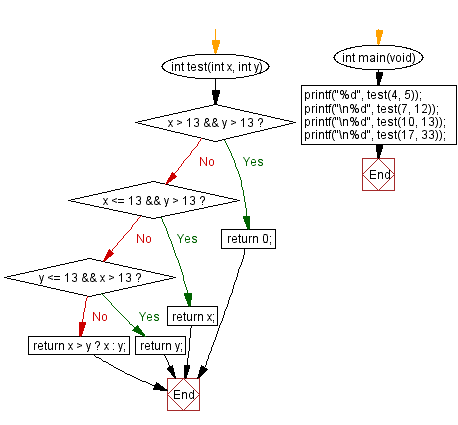﻿ C Program: Find the value closest to 13 from 2 integers# C Exercises: Check two given integers and return the value whichever value is nearest to 13 without going over

## C-programming basic algorithm: Exercise-32 with Solution

Write a C program to check two given integers and return the value whichever value is nearest to 13 without going over. Return 0 if both numbers go over.

C Code:

``````#include <stdio.h>
#include <stdlib.h>

int main(void){
printf("%d",test(4, 5));
printf("\n%d",test(7, 12));
printf("\n%d",test(10, 13));
printf("\n%d",test(17, 33));
}
int test(int x, int y)
{
if (x > 13 && y > 13) return 0;
if (x <= 13 && y > 13) return x;
if (x <= 13 && y > 13) return y;
return x > y ? x : y;
}
``````

Sample Output:

```5
12
13
0
```

Pictorial Presentation:Flowchart:C Programming Code Editor:

What is the difficulty level of this exercise?

Test your Programming skills with w3resource's quiz.

﻿

## C Programming: Tips of the Day

Why doesn't a+++++b work?

printf("%d",a+++++b); is interpreted as (a++)++ + b according to the Maximal Munch Rule!.

++ (postfix) doesn't evaluate to an lvalue but it requires its operand to be an lvalue.

! 6.4/4 says the next preprocessing token is the longest sequence of characters that could constitute a preprocessing token"

Ref : https://bit.ly/3fdldUT